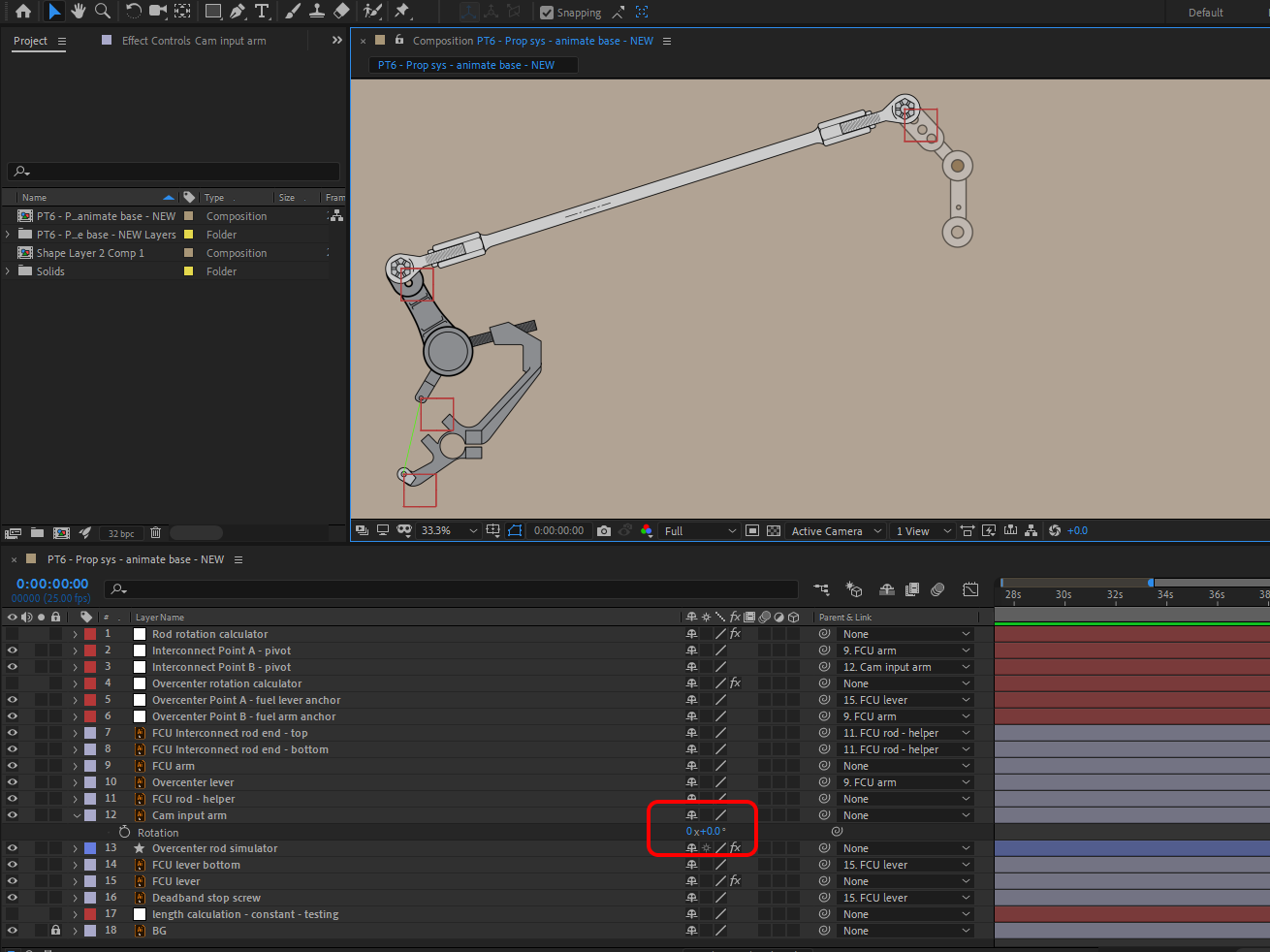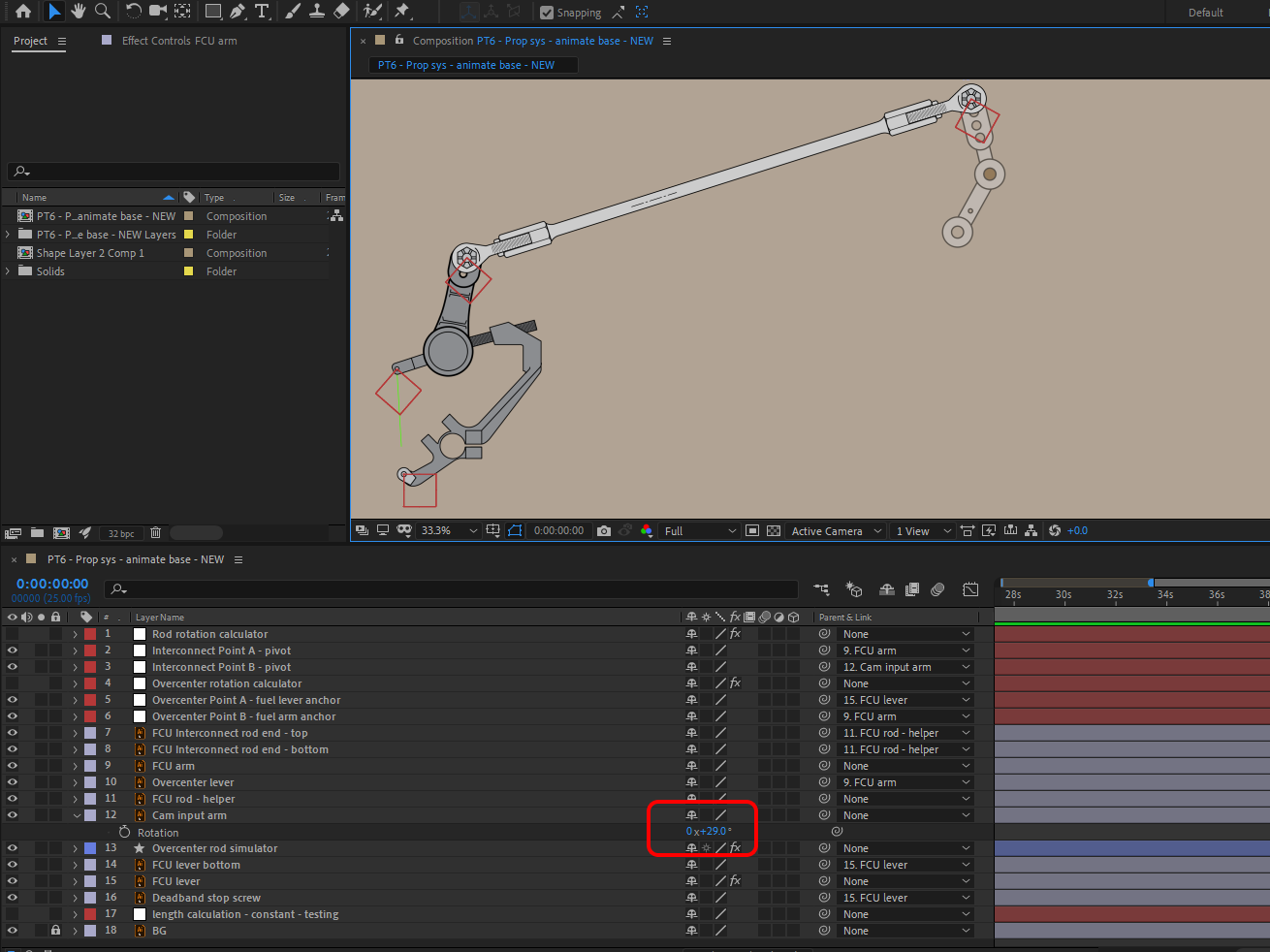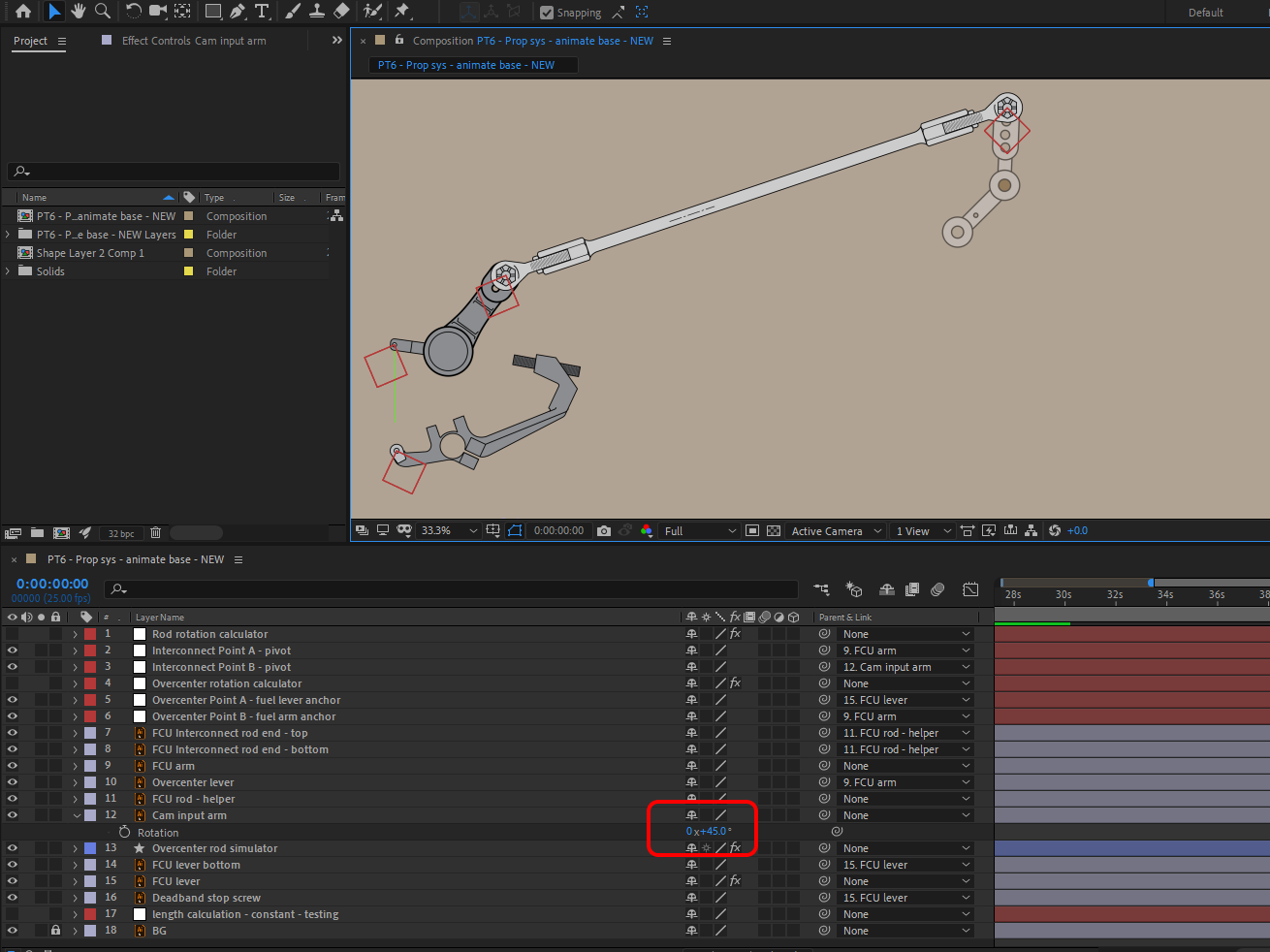ADOBE AFTER EFFECTS: Forum Expressions Tutorials Creative Cloud

Two interconnected points of rotation

FAQ   •   VIEW ALLTwo interconnected points of rotation on Feb 3, 2019 at 5:44:47 pm

Hi all,
Need a bit of help on this animation of "two interconnected points of rotation".
Here is a photo for visual aid:It's basically two mechanical "levers" that are connected together by a rod. The right one is the input (green arm), which controls the "fuel arm" to the left.

I managed to get the rod to rotate properly with the rotation of the input (and the rod automatically faces itself toward the anchor point of the fuel arm), but I can't figure out a way to calculate the proper angle for the fuel arm rotation (relative to input rotation angle).

For example: If green arm is rotated by 30 degrees, the resultant angle of fuel arm rotation is roughly 42.5 degrees. I'd like to make an expression for this so it's done automatically without me constantly having to manually rotate the fuel arm after rotating the green input arm.

Note: The mechanism is physically limited in the range of -40 degrees to +60 degrees, so the green input arm will never be outside of that range.

For those wondering, it's the fuel control mechanism of a PT6 turbine engine.
The top part of the image is the "zero" point. All rotation are done from this initial point. So ultimately it's the rotation angle of line "E" that I'm after, in relation to line "F" rotation.

Thanks for any suggestions!Re: Two interconnected points of rotationon Feb 4, 2019 at 7:54:30 am

It can be done with an iteration algorithm:

In my implementation the rod is attached to Arms 1 and 2 at Nulls 1 and 2;
Arm 2 is Input.
Arm 1 is Fuel.

This expression for Arm 1 Rotation approximates the Rotation Angle of Arm 1 till the distance between attachment points [almost] equals the length of the Rod:

//computes the difference between (target) Rod's length and a length you get when dependent Arm's rotation is alpha
var deltaRodLength = function(alpha){
R1 = thisComp.layer("Animation Controls").effect("D1")("Slider")/ 2;
arm1 = thisComp.layer("Arm 1");
arm2 = thisComp.layer("Arm 2");
//point at which Rode is attached to Arm 2
null2Pos = arm2.toWorld(thisComp.layer("Null 2").position);

//Rode length
targetRodLength = thisComp.layer("Animation Controls").effect("Rod Length")("Slider");
currentRodLength = length([R1*Math.cos(alpha), -R1*Math.sin(alpha)]+arm1.position, null2Pos);

return currentRodLength - targetRodLength;
}

a = 0
b = Math.PI;
err = 0.001

//iteration algo
i=0
while (Math.abs(deltaRodLength((a+b)/ 2)) >=err){
if (deltaRodLength((a+b)/ 2) > 0){b = (a+b)/ 2;}
if (deltaRodLength((a+b)/ 2) < 0){a = (a+b)/ 2;}
if (deltaRodLength((a+b)/ 2) == 0){
a = (a+b)/ 2;
b = (a+b)/ 2;
}

//this prevents AE from freezing when no solution exists
i++;
if (i>200) {break;};
}

Surprisingly neat expression.
Of cause, it can also been done with an explicit trigonometric formula for the Fuel Arm angle, but it's quite hideous.Re: Two interconnected points of rotationon Feb 5, 2019 at 5:05:35 am

Although I haven't been able to modify your expression to work correctly in my composition (yet), I'm really impressed with how quickly you came up with that! Thank you!

I modified it to try to make it work with my layer names and dimensions, but I can't figure it out.

Here's what I have so far:

Arm 1 = "FCU arm"
Arm 2 = "Cam input arm"

Rod attaches to FCU arm (arm1) at Null 1 ("Interconnect Point A - pivot")
Road attaches to Cam input arm (arm2) at Null 2 ("Interconnect Point B - pivot")

Parenting:
Null 1 parent is FCU arm
Null 2 parent is Cam input arm

Rod position expression:
B = thisComp.layer("Interconnect Point B - pivot");
B.toWorld(B.anchorPoint);

Null 3 ("Rod Point C") is parented to the rod (where it attaches to FCU arm (arm1).
Used to calculate rod length.

Photo below:Link to AE file below, if you want to have a closer look

Appreciate any further help,
Thank you

//computes the difference between (target) Rod's length and a length you get when dependent Arm's rotation is alpha
var deltaRodLength = function(alpha){
arm1 = thisComp.layer("FCU arm");
arm2 = thisComp.layer("Cam input arm");
rod = thisComp.layer("FCU Interconnect rod");
//point at which Rod is attached to Arm 1
null1Pos = arm1.toWorld(thisComp.layer("Interconnect Point A - pivot").position);
//point at which Rod is attached to Arm 2
null2Pos = arm2.toWorld(thisComp.layer("Interconnect Point B - pivot").position);
//point at which Rod is attached to Arm 1, parented to rod
null3Pos = rod.toWorld(thisComp.layer("Rod Point C").position);
R1 = length(thisComp.layer("FCU arm").position, null1Pos);

//Rod length
targetRodLength = length(null3Pos, null2Pos);
currentRodLength = length([R1*Math.cos(alpha), -R1*Math.sin(alpha)]+arm1.position, null2Pos);

return currentRodLength - targetRodLength;
}

a = 0
b = Math.PI;
err = 0.001

//iteration algo
i=0
while (Math.abs(deltaRodLength((a+b)/ 2)) >=err){
if (deltaRodLength((a+b)/ 2) > 0){b = (a+b)/ 2;}
if (deltaRodLength((a+b)/ 2) &lt; 0){a = (a+b)/ 2;}
if (deltaRodLength((a+b)/ 2) == 0){
a = (a+b)/ 2;
b = (a+b)/ 2;
}

//this prevents AE from freezing when no solution exists
i++;
if (i>200) {break;};
}Re: Two interconnected points of rotationon Feb 5, 2019 at 7:31:48 am

The implementation is generally fine as is.
If you put the Rod layer ("FCU Interconnect Rod") beneath the FCU arm layer, it will work like intended, as I presume:

Switching layers' order is not a proper solution though, the problem is that you have a cross reference:

Rod -(expression)-> A
A -(child)-> FCU arm
FCU arm -(expression)-> C
C -(child) -> Rod

Rod -(expression)-> A -(child)-> FCU arm -(expression)-> C -(child) -> Rod

In this case Gd only knows in what order AE will render and what output you'll get.

If Rod is above FCU arm you get this:If Rod is below FCU arm you (may) get:The Rod image is in the right place but null and source rectangle are not.
This can be cured by cache cleaning but may also lead to various bugs in the future.

Bottom line, you would probably wanna find another way to define the Rod length (maybe just a constant), thus breaking the reference loop at C-point. Namely, getting rid of this reference:
//point at which Rod is attached to Arm 1, parented to rod
null3Pos = rod.toWorld(thisComp.layer("Rod Point C").position);Re: Two interconnected points of rotationon Feb 9, 2019 at 10:43:54 pm

Oleg,

Thank you much for the help! I got that interconnect working with your expressions and suggestions.
I decided to use the constant rod length.

I am using your same method to rotate another 3rd lever, attached to the FCU arm. Still needs some tweaking, but I'm very close!
AE Files:

If you rotate the green "cam input lever" you can see how it works so far.
Seems to rotate the 3rd lever only after 30 degrees on the green arm.Re: Two interconnected points of rotationon Feb 11, 2019 at 7:54:27 am

I see you've switched to AE CC 2019. Bear in mind that it has an updated expression engine and is much more strict on syntax and
I've only tested this code in previous versions. Though, I believe, I was careful with syntax, still some issues may rise when running this code in CC 2019.Re: Two interconnected points of rotationon Sep 14, 2019 at 5:52:30 pm

Hi Oleg,

Apologies for bringing back such an old thread, but I wanted to give this another attempt - I never did get that second lever working properly. The second lever only rotates after approximately +29 degrees of input from the main input arm.

In following images, the green path is the "rod".

0 degrees:29 degrees45 degreesWhat do you think is the cause of the gap between 0 and 29 degrees?
Appreciate any help, thank you!

Below are the project files in CC 2019:

I can add the CC 2018 version if needed..

//computes the difference between (target) Rod's length and a length you get when dependent Arm's rotation is alpha
var deltaRodLength = function(alpha){
arm1 = thisComp.layer("FCU lever");
arm2 = thisComp.layer("FCU arm");
rod = thisComp.layer("Overcenter rod simulator");
//point at which Rod is attached to Arm 1
null1Pos = arm1.toWorld(thisComp.layer("Overcenter Point A - fuel lever anchor").position);
//point at which Rod is attached to Arm 2
null2Pos = arm2.toWorld(thisComp.layer("Overcenter Point B - fuel arm anchor").position);
R1 = length(thisComp.layer("FCU lever").position, null1Pos);
//Rod length
targetRodLength = 240.81;
currentRodLength = length([R1*Math.cos(alpha), -R1*Math.sin(alpha)]+arm1.position, null2Pos);

return currentRodLength - targetRodLength;
}

a = 0
b = Math.PI;
err = 0.001

//iteration algo
i=0
while (Math.abs(deltaRodLength((a+b)/2)) >=err){
if (deltaRodLength((a+b)/2) > 0){b = (a+b)/2;}
if (deltaRodLength((a+b)/2) &lt; 0){a = (a+b)/2;}
if (deltaRodLength((a+b)/2) == 0){
a = (a+b)/2;
b = (a+b)/2;
}

//this prevents AE from freezing when no solution exists
i++;
if (i>100) {break;};
}